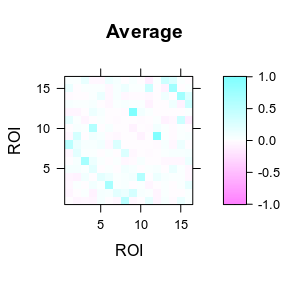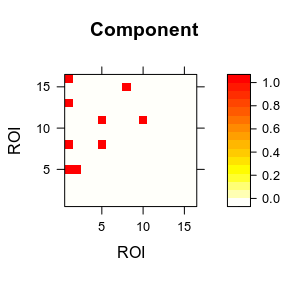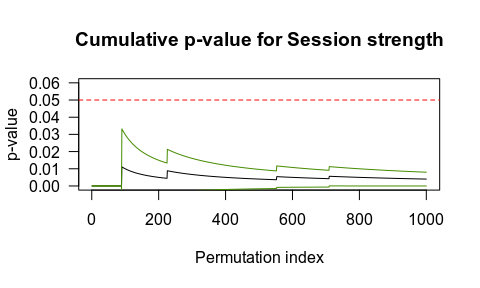# Network-Based R-statistics for mixed-effects models

This vignette documents the implementation of NBR 0.1.3 for linear mixed effect (LME) models.

We will analyze the voles dataset, which contains a matrix of 96 rows (sessions) and 123 columns (variables). The first three variables include phenotypic information of the subjects/sessions (1: subject ID; 2: Sex; 3: Session 1-3), the remaining 120 variables include the upper triangle edges of a network of 16 brain regions (fMRI functional connectivity).

NOTE: for more detail of the dataset execute help(voles).

library(NBR)
data("voles")
brain_labs <- NBR:::voles_roi
dim(voles)
#>   96 123
#>    id Sex Session     ACC.AON     ACC.BLA     AON.BLA    ACC.BNST     AON.BNST
#> 1 F01   F     1st -0.28686306 -0.40153834 -0.14665024 -0.08307386  0.045431614
#> 2 F01   F     2nd -0.31489561  0.01090166 -0.05047274 -0.07112861  0.005440684
#> 3 F01   F     3rd -0.01423683 -0.07658247 -0.01224975 -0.21711713 -0.048013603
#> 4 F02   F     1st -0.17290194 -0.18256430  0.09607568  0.03990079 -0.116842983
#> 5 F02   F     2nd -0.29984543 -0.17029284 -0.19706318 -0.12662968 -0.039984274
#> 6 F02   F     3rd          NA          NA          NA          NA           NA

Here we can obtain the corresponding pairwise interaction of the brain network for each edge.

nnodes <- length(brain_labs)
tri_pos <- which(upper.tri(matrix(nrow = nnodes, ncol = nnodes)), arr.ind = T)
#>      row col
#> [1,]   1   2
#> [2,]   1   3
#> [3,]   2   3
#> [4,]   1   4
#> [5,]   2   4
#> [6,]   3   4

IT’S VERY IMPORTANT that the order of the columns containing the network data matches with the order of the upper triangle of the network matrix.

Let’s plot the average network with lattice::levelplot.

library(lattice)
avg_mx <- matrix(0, nrow = nnodes, ncol = nnodes)
avg_mx[upper.tri(avg_mx)] <- apply(voles[-(1:3)], 2, function(x) mean(x, na.rm=TRUE))
avg_mx <- avg_mx + t(avg_mx)
# Set max-absolute value in order to set a color range centered in zero.
flim <- max(abs(avg_mx))
levelplot(avg_mx, main = "Average", ylab = "ROI", xlab = "ROI",
at = seq(-flim, flim, length.out = 100))The next step is to check the dataset to be tested edgewise. In this case we are going to test if the variables Sex, Session, and their interaction (Sex:Session) have any effect related to the brain networks. Since every subject was assessed in three different sessions, we should add the intercept and the Session term as random effects adding the random formula ~ 1+Session|id, where id accounts for the subject label.

set.seed(18900217)
before <- Sys.time()
library(nlme)
nbr_result <- nbr_lme_aov(net = voles[,-(1:3)],
nnodes = 16,
idata = voles[,1:3],
nperm = 5,
mod = "~ Session*Sex",
rdm = "~ 1+Session|id",
na.action = na.exclude)
after <- Sys.time()
show(after-before)

Although five permutations is quite low to obtain a proper null distribution, we can see that they take several seconds to be performed. So we suggest paralleling to multiple CPU cores with the cores argument.

set.seed(18900217)
before <- Sys.time()
library(nlme)
library(parallel)
nbr_result <- nbr_lme_aov(
net = voles[,-(1:3)],
nnodes = 16,
idata = voles[,1:3],
nperm = 1000,
nudist = T,
mod = "~ Session*Sex",
rdm = "~ 1+Session|id",
cores = detectCores(),
na.action = na.exclude
)
after <- Sys.time()
show(after-before)

This may elapse approximately 15 minutes in an Intel(R) Core(TM) i7-8700 CPU @ 3.20GHz with 12 cores. But we can load those results instead of running them again.

nbr_result <- NBR:::voles_nbr
show(nbr_result$fwe) #>$Session
#>   Component ncomp ncompFWE     strn strnFWE
#> 1         1     9    0.135 28.22975   0.004
#>
#> $Sex #> Component ncomp ncompFWE strn strnFWE #> 1 1 2 0.711 4.0351647 0.801 #> 2 2 1 0.980 0.7516185 0.999 #> 3 3 3 0.316 5.2619146 0.587 #> #>$Session:Sex
#>    Component ncomp ncompFWE       strn strnFWE
#> 1          1     1    0.954 2.78159791   0.846
#> 2          2     1    0.954 0.07695101   0.995
#> 3          3     2    0.821 2.13757157   0.905
#> 4          4     1    0.954 0.77359511   0.985
#> 6          6     1    0.954 0.20354124   0.994
#> 15        15     1    0.954 1.77582539   0.943

If we observed the Family-Wise Error (FWE) probabilities of the observed components, only the component 1 in the Session term is lower than the nominal alpha of p < 0.05. The table shows the probabilities associated with: 1) the number of connected edges, and 2) the sum of the strength of the edges. In this case, we will use the sum of strengths, but you can choose depending on your research question.

Let’s display the FWE-corrected component.

# Plot significant edges
edge_mat <- array(0, dim(avg_mx))
edge_mat[nbr_result$components$Session[,2:3]] <- 1
levelplot(edge_mat, col.regions = rev(heat.colors(100)),
main = "Component", ylab = "ROI", xlab = "ROI")Lastly, if we are not sure if 1000 permutations are enough we can plot the cumulative p-value (black line) with its corresponding binomial marginal error (green lines). To do so, you just need to set TRUE for the return null distribution argument (nudist).

null_ses_str <- nbr_result$nudist[,2] # Null distribution for Session strength obs_ses_str <- nbr_result$fwe\$Session[,4] # Observed Session strength
nperm <- length(null_ses_str)
cumpval <- cumsum(null_ses_str >= obs_ses_str)/(1:nperm)
# Plot p-value stability
plot(cumpval, type="l", ylim = c(0,0.06), las = 1,
xlab = "Permutation index", ylab = "p-value",
main = "Cumulative p-value for Session strength")
abline(h=0.05, col="red", lty=2)
lines(cumpval-mepval, col = "chartreuse4")